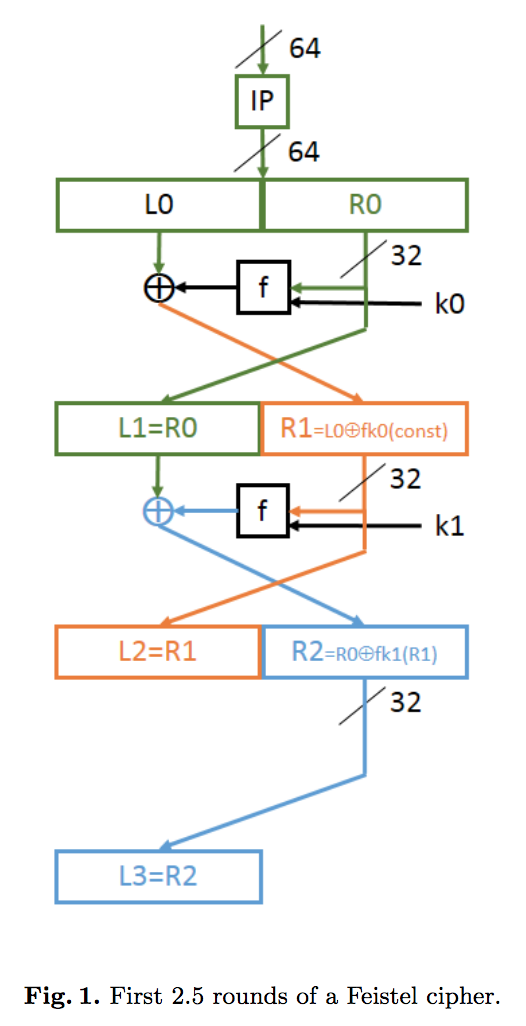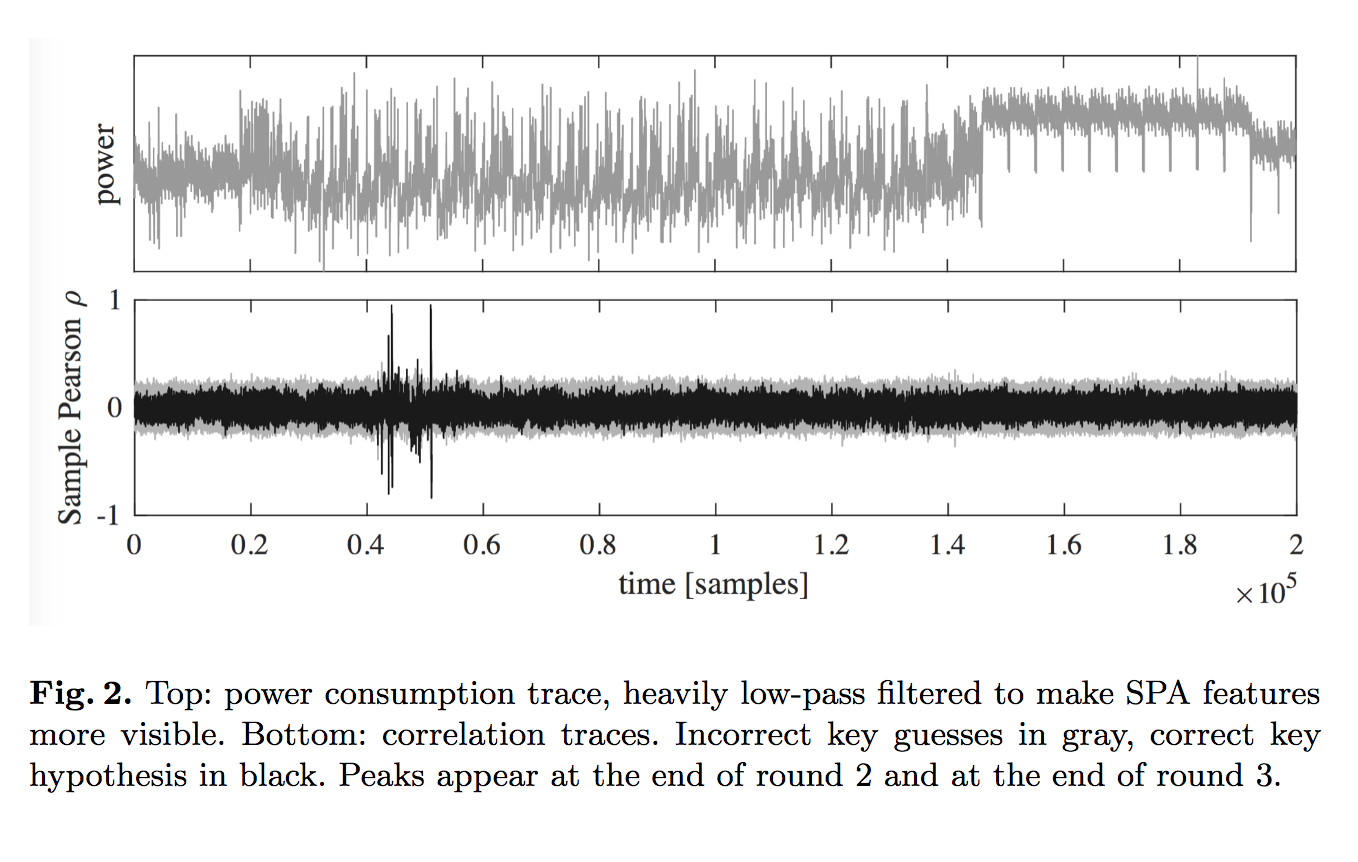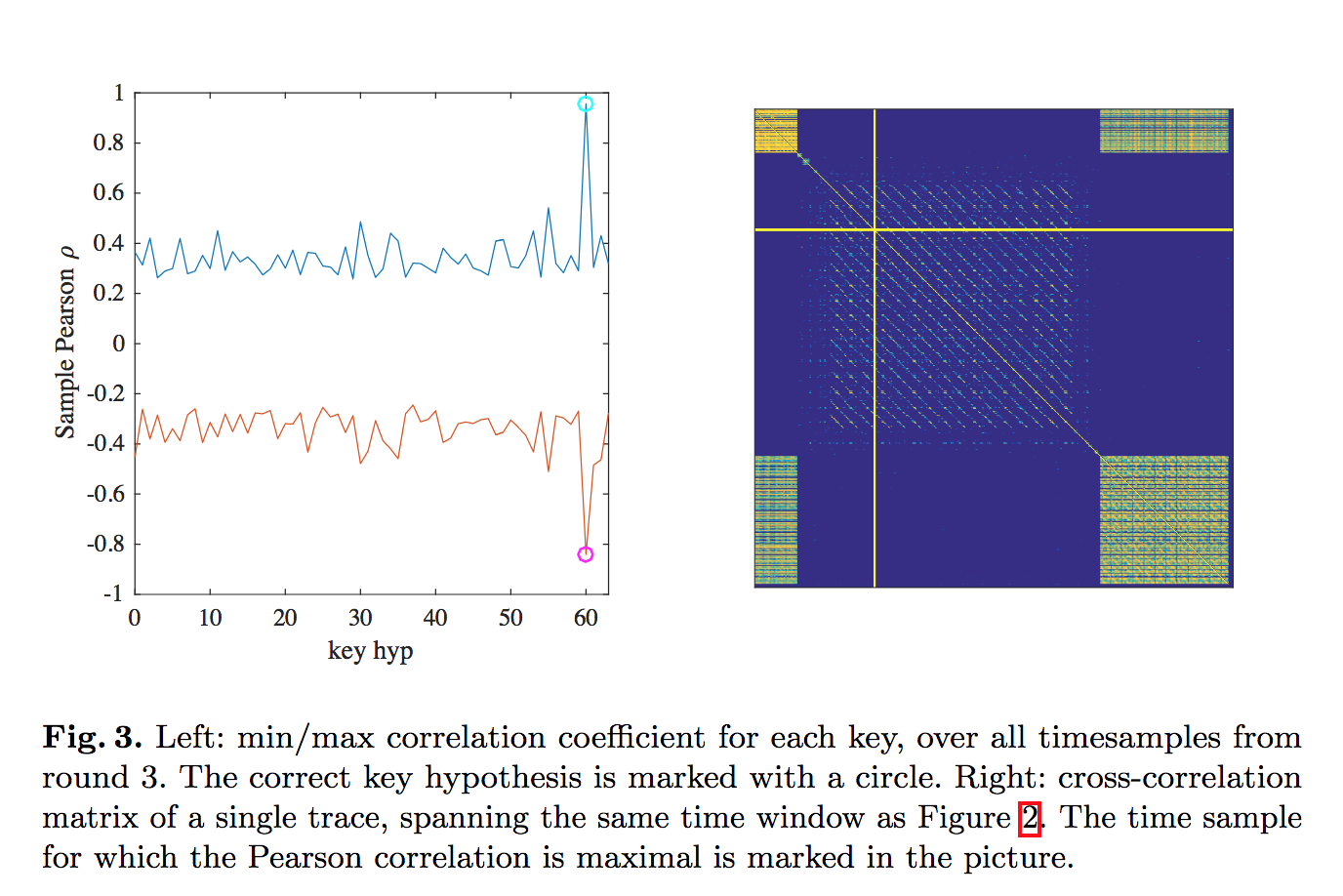Straight DPA attacks target the outer rounds of a cipher: either the first one (if you know the input) or the last one (if you know the output). In a Feistel construction, you can easily DPA the second round. Things are messier if you want to target inner rounds.

Here we describe a simple, cute DPA attack on the third round output of a Feistel. There are much more advanced attacks that target fourth and even deeper rounds of a Feistel; this attack is on the other hand very simple (just assumes DPA works, no fancy leakage assumptions) and very fun. The full details are in our paper:

A first-order chosen-plaintext DPA attack on the third round of DES

O. Reparaz, B. Gierlichs

CARDIS 2017On the left you have the three first rounds of a Feistel. In the case of DES, the 64-bit input is placed into two 32-bit words $$(L_0,R_0)$$ and then we crank the cipher. In the following, we assume we only get side-channel leakage from $$(L_3,R_3)$$. This is a good approximation to what happens if the first two rounds are well protected (for example, by masking).

Step 1. Step 1 consists of a DPA attack targeting $$L_3$$ with chosen input: vary $$L_0$$ and fix $$R_0$$. When you craft your inputs like this, you’re deactivating the first round. The first round output stays constant, so in the expression $$L_1=L_0 \oplus F_{k_1}(R_0)$$ the $$F_{k_1}(R_0)$$ term is unknown but constant.

We target leakage from $$L_3$$ to recover $$k_1\oplus F_{k_0}(R_0)$$. (We’re ignoring the expansion function to make the explanation easier.) Since it is a Feistel, diffusion only affects half of the state per round. So using leakage corresponding to input of round 4 we can recover second round subkey. So far we recovered the second round subkey $$k_1$$ plus an unknown offset $$F_{k_0}(R_0)$$. We have no idea about this offset $$F_{k_1}(R_0)$$. All we know is that it’s a constant.

Step 2. To untangle the two terms, we perform a super basic key-recovery differential attack on 1-round of a Feistel. The basic idea is that the output difference $$\Delta = F_{k_0}(R_0) \oplus F_{k_0}(R_0')$$ after one round leaks an awful lot information on the key $$k_0$$. So we repeat step 1 (picking a different fixed $$R'_0$$) to collect an extra $$k_2 \oplus F_{k_1}(R_0')$$, derive the differential $$\Delta$$ (the $$k_2$$ term cancels out) and then recover the key $$k_0$$. We need to collect a few (2 or 3) different $$\Delta$$ (by repeating step 1 a few times). From here on, everything falls down. You recover $$k_0$$, then you can plug it to solve for $$k_1$$ and you’re basically done, since you recovered two consecutive round keys and you can invert the key schedule.Proof of concept. We implemented this attack end-to-end. The details are https://eprint.iacr.org/2017/1257.pdf. We exploit leakage only from the third round and perform the simple differential attack yielding just 4 candidates for $$k_0$$.